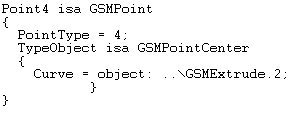# GSMPoint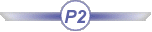## Definition:

A GSMPoint is a point:

• generated by the Generative Shape Design product
• available in the BasicWireFrame Package.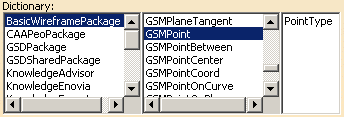## Attributes:

###### PointType

A point is defined by its type. The attribute to use is PointType. The syntax to be used is: PointType = i, i corresponding to the type of point that you want to create.
Please find below a table listing the existing types of points that you can create and the digit to indicate.
 Plane Type in GSD Plane Type in the Package Corresponding digit Coordinates GSMPointCoord 0 On surface GSMPointOnSurface 1 On curve GSMPointOnCurve 2 On plane GSMPointOnPlane 3 Circle center GSMPointCenter 4

As mentionned above, you may create 5 different point sub-types. Please find below a description of each sub-type, as well as its attributes and the syntax to use.

### Coordinates (GSMPointCoord)

The sub-type to be used in this case is GSMPointCoord which enables you to create a coordinate point. The following attributes are available for this sub-type:

 RefPoint (Reference point, optional). If specified, x, y, and z are indicated in a mark whose origin is this reference point. X (First coordinate) Y (Second coordinate) Z (Third coordinate)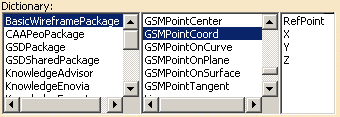These attributes can be combined as follows:

Combination
• RefPoint (Reference point, optional)
• X which is defined by the syntax below:
`X = 10mm;`
• Y which is defined by the syntax below:
`Y = 10mm;`
• Y which is defined by the syntax below:
`Z = 10mm;`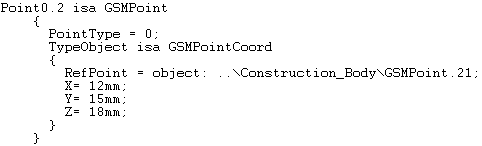### On surface (GSMPointOnSurface)

The sub-type to be used in this case is GSMPointOnSurface which creates a point on a plane. The following attributes are available for this sub-type:

 Direction: Element taking its orientation as reference direction or a plane taking its normal as reference direction RefPoint: Reference point. By default, the surface middle point is taken as reference. Support: Surface where the point is to be created. Values: Distance along the reference direction used to display a point.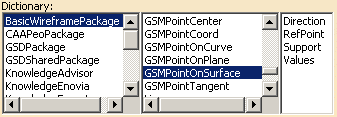These attributes can be combined as follows:

Combination
• Direction which is defined by the syntax below:
`Direction = object: ..\Line.1;`
• Support which is defined by the syntax below:
`Support= object: ..\Extrude.1;`
• Values which is defined by the syntax below:
`Values = 12mm;`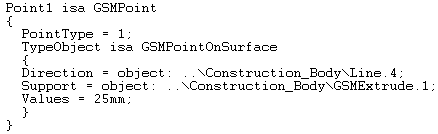### On curve (GSMPointOnCurve)

The sub-type to be used in this case is GSMPointOnCurve which enables you to create a point on a curve. The following attributes are available for this sub-type:

 Boundary: Not available. RefPoint: Reference point. If not specified, it is the extremity of the curve. Support: Curve Values: Distance between the reference point and this point.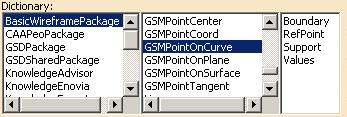Combination
• Refpoint which is defined by the syntax below:
`RefPoint= object: ..\Point.1;`
• Support which is defined by the syntax below:
`Support = object: ..\Line.1;`
• Values which is defined by the syntax below:
`Values = 12mm;`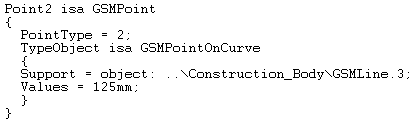### On plane (GSMPointOnPlane)

The sub-type to be used in this case is GSMPointOnPlane. It creates a point on a plane. The following attributes are available for this sub-type:

 Direction (optional). When specified, indicates the direction H: Vector. RefPoint: point used to define a reference for computing coordinates in the plane. Support: Plane on which the point will be created. V: Vector.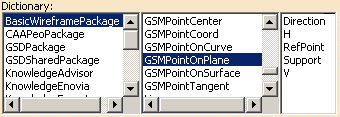The attributes must be used as follows:

Combination
• Direction which is defined by the syntax below:
`Direction = object: ..\Line.1;`
• H which is defined by the syntax below:
`H = 150mm;`
• RefPoint which is defined by the syntax below:
`RefPoint= object: ..\Point.1;`
• Support which is defined by the syntax below:
`Support = object: 'xy plane'`
• V which is defined by the syntax below:
`V = 150mm;`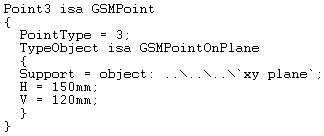### Circle Center (GSMPointCenter)

The sub-type to be used in this case is GSMPointCenter which enables you to define the center of a circle.

 Curve: circle, circular arc, or ellipse.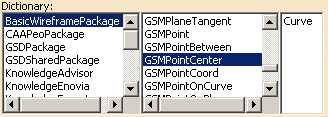This attribute is to be used as follows:

Combination
• Curve which is defined by the syntax below:
`Curve`` = object: ..\Extrude.1;`